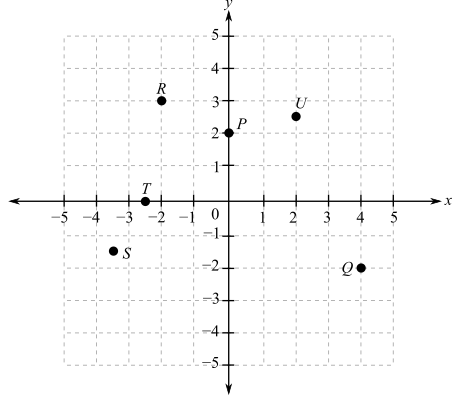Chapter 0.7, Problem 1E

Chapter
Section
Textbook Problem

Referring to the following figure, determine the coordinates of the indicated points as accurately as you can.To determine

The co-ordinates of the indicated points in the provided graph,Explanation

Given Information:

The provided graph is,

Consider the provided graph,

Start with the alphabetical order, the provided points are P, Q, R, S, T and U.

First take point P,

The point P has height of 2 units, so its coordinates are (0,2).

Consider next point R,

Now from the origin, drop a vertical line of x=2 and horizontal line of y=3. Therefore, the coordinates are (2,3).

Consider next point U,

Now from the origin drop a vertical line of x=2 and horizontal line of y=2.5. Therefore, the coordinates are (2,2.5).

Similarly, for point Q, S, and T,

The coordinates are Q(4,2), S(3

Still sussing out bartleby?

Check out a sample textbook solution.

See a sample solution

The Solution to Your Study Problems

Bartleby provides explanations to thousands of textbook problems written by our experts, many with advanced degrees!

Get Started

In Exercises 69-74, rationalize the numerator. 74. x2y32x

Applied Calculus for the Managerial, Life, and Social Sciences: A Brief Approach

Multiply the following numbers and verify your answers. 4.

Contemporary Mathematics for Business & Consumers

The area of the shaded region is given by:

Study Guide for Stewart's Multivariable Calculus, 8th